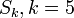# Difference between revisions of "Symmetric group:S5"

View a complete list of particular groups (this is a very huge list!)[SHOW MORE]

## Definition

The symmetric group$S_5$ is defined in the following equivalent ways:

## Arithmetic functions

Function Value Similar groups Explanation
order (number of elements, equivalently, cardinality or size of underlying set) 120 groups with same order As$\! S_k, k = 5:$$\! 5! = 5 \cdot 4 \cdot 3 \cdot 2 \cdot 1 = 120$
exponent 60 groups with same order and exponent | groups with same exponent As$\! S_k, k = 5:$$\! \operatorname{lcm} \{1,2,3,4,5 \} = 60$
derived length -- not a solvable group.
nilpotency class -- not a nilpotent group.
Frattini length 1 groups with same order and Frattini length | groups with same Frattini length Frattini-free group: intersection of maximal subgroups is trivial.
minimum size of generating set 2 groups with same order and minimum size of generating set | groups with same minimum size of generating set$\! (1,2), (1,2,3,4,5)$; see also symmetric group on a finite set is 2-generated
subgroup rank of a group 2 groups with same order and subgroup rank of a group | groups with same subgroup rank of a group
max-length of a group 5 groups with same order and max-length of a group | groups with same max-length of a group
number of subgroups 156 groups with same order and number of subgroups | groups with same number of subgroups
number of conjugacy classes 7 groups with same order and number of conjugacy classes | groups with same number of conjugacy classes As$S_k, k = 5$: the number of conjugacy classes is$p(k) = p(5) = 7$, where$p$ is the number of unordered integer partitions. See also cycle type determines conjugacy class
number of conjugacy classes of subgroups 19 groups with same order and number of conjugacy classes of subgroups | groups with same number of conjugacy classes of subgroups

## Group properties

COMPARE AND CONTRAST: Want to know more about how this group compares with symmetric groups of other degrees? Read contrasting symmetric groups of various degrees.
Property Satisfied Explanation Comment
Abelian group No$(1,2)$,$(1,3)$ don't commute$S_n$ is non-abelian,$n \ge 3$.
Nilpotent group No Centerless: The center is trivial$S_n$ is non-nilpotent,$n \ge 3$.
Metacyclic group No No cyclic normal subgroup$S_n$ is not metacyclic,$n \ge 4$.
Supersolvable group No No cyclic normal subgroup$S_n$ is not supersolvable,$n \ge 4$.
Solvable group No The subgroup$A_5$ is simple non-abelian$A_n$ is simple and hence$S_n$ not solvable,$n \ge 5$.
T-group Yes
HN-group Yes
Complete group Yes Centerless and every automorphism's inner Symmetric groups are complete except the ones of degree$2,6$.
Monolithic group Yes Monolith is the alternating group All symmetric groups are monolithic;$n=4$ is the only case the monolith is not the alternating group.
One-headed group Yes The alternating group is the unique maximal normal subgroup True for all$n > 1$.

## Elements

### Upto conjugacy

For convenience, we take the underlying set to be$\{ 1,2,3,4,5 \}$.

There are seven conjugacy classes, corresponding to the unordered integer partitions of$5$ (for more information, refer cycle type determines conjugacy class). We use the notation of the cycle decomposition for permutations:

Partition Verbal description of cycle type Representative element with the cycle type Size of conjugacy class Formula calculating size Even or odd?
1 + 1 + 1 + 1 + 1 five fixed points$()$ -- the identity element 1$\! \frac{5!}{(1)^5(5!)}$ even
2 + 1 + 1 + 1 transposition: one 2-cycle, three fixed point$(1,2)$ 10$\! \frac{5!}{(2)(1)^3(3!)}$, also$\binom{5}{2}$ in this case odd
3 + 1 + 1 one 3-cycle, two fixed points$(1,2,3)$ 20$\! \frac{5!}{(3)(1)^2(2!)}$ even
2 + 2 + 1 double transposition: two 2-cycles, one fixed point$(1,2)(3,4)$ 15$\! \frac{5!}{(2)^2(2!)(1)}$ even
4 + 1 one 4-cycle, one fixed point$(1,2,3,4)$ 30$\! \frac{5!}{(4)(1)}$ odd
3 + 2 one 3-cycle, one 2-cycle$(1,2,3)(4,5)$ 20$\! \frac{5!}{(3)(2)}$ odd
5 one 5-cycle$(1,2,3,4,5)$ 24$\! \frac{5!}{5}$ even

### Upto automorphism$S_5$ is a complete group: in particular, every automorphism of the group is inner. Thus, the equivalence classes under automorphisms are the same as the conjugacy classes.

## Endomorphisms

### Automorphisms

Since$S_5$ is a complete group, it is isomorphic to its automorphism group, where each element of$S_4$ acts on$S_4$ by conjugation.

### Endomorphisms$S_5$ admits three kinds of endomorphisms (that is, it admits more endomorphisms, but any endomorphism is equivalent via an automorphism to one of these three):

• The endomorphism to the trivial group
• The identity map
• The endomorphism to a group of order two, given by the sign homomorphism

## Subgroups

Further information: Subgroup structure of symmetric group:S5

## GAP implementation

### Group ID

This finite group has order 120 and has ID 34 among the groups of order 120 in GAP's SmallGroup library. For context, there are 47 groups of order 120. It can thus be defined using GAP's SmallGroup function as:

SmallGroup(120,34)

For instance, we can use the following assignment in GAP to create the group and name it$G$:

gap> G := SmallGroup(120,34);

Conversely, to check whether a given group$G$ is in fact the group we want, we can use GAP's IdGroup function:

IdGroup(G) = [120,34]

or just do:

IdGroup(G)

to have GAP output the group ID, that we can then compare to what we want.

### Other descriptions

The group can also be defined using GAP's SymmetricGroup function as:

SymmetricGroup(5)